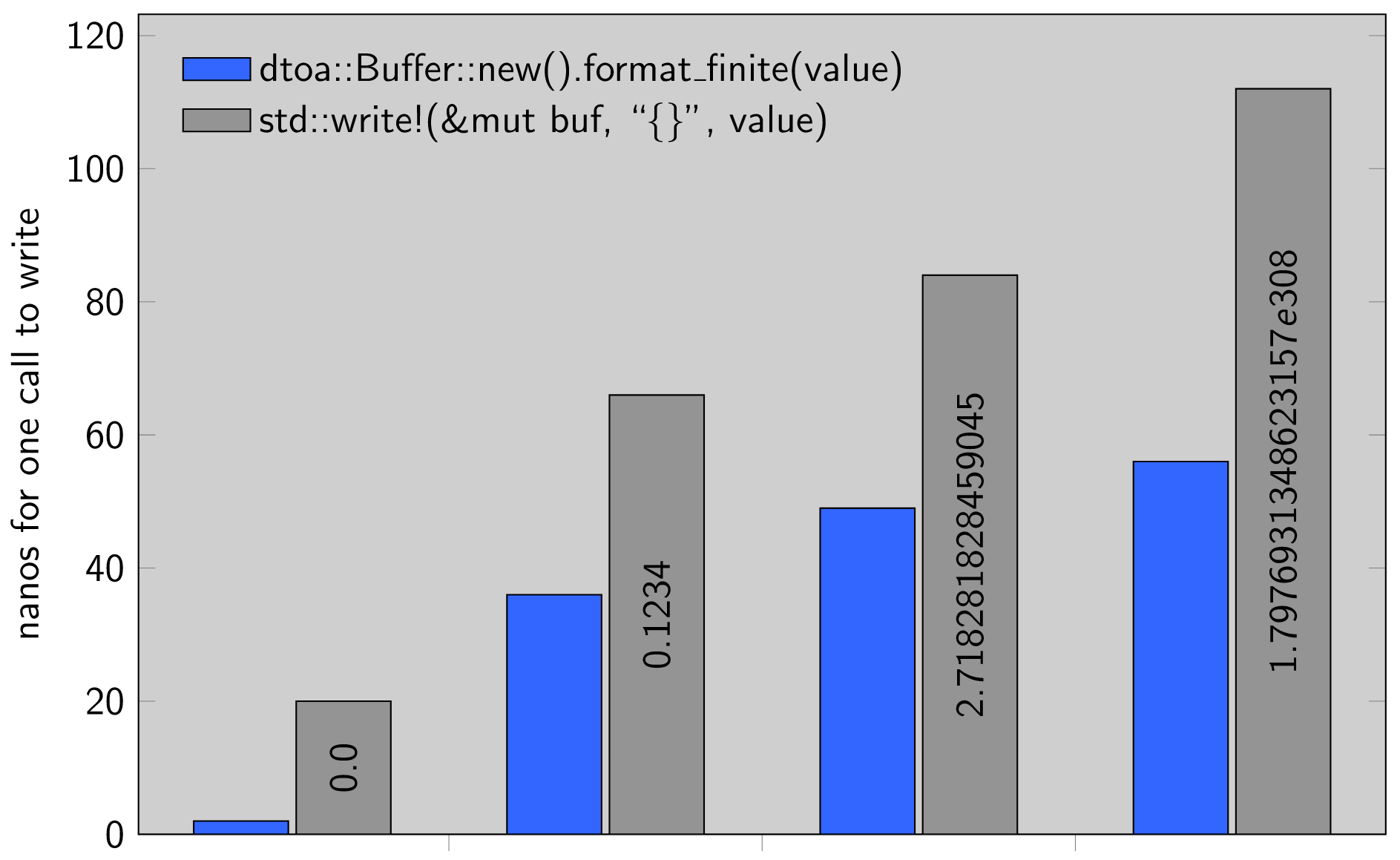# dtoa 0.4.2

Fast functions for printing floating-point primitives to an io::Write

# dtoa

This crate provides fast functions for printing floating-point primitives to an `io::Write`. The implementation is a straightforward Rust port of Milo Yip's C++ implementation dtoa.h. The original C++ code of each function is included in comments.

See also `itoa` for printing integer primitives.

## Performance (lower is better)## Functions

``````extern crate dtoa;

// write to a vector or other io::Write
let mut buf = Vec::new();
dtoa::write(&mut buf, 2.71828f64)?;
println!("{:?}", buf);

// write to a stack buffer
let mut bytes = [b'\0'; 20];
let n = dtoa::write(&mut bytes[..], 2.71828f64)?;
println!("{:?}", &bytes[..n]);
``````

The function signature is:

``````fn write<W: io::Write, V: dtoa::Floating>(writer: W, value: V) -> io::Result<()>
``````

where `dtoa::Floating` is implemented for `f32` and `f64`. The return value gives the number of bytes written.

## Dependency

Dtoa is available on crates.io. Use the following in `Cargo.toml`:

``````[dependencies]
dtoa = "0.4"
``````